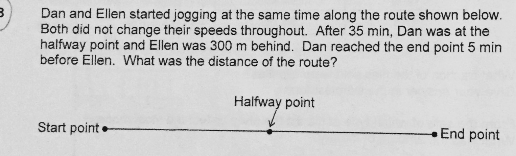# QuestionAnyone help me with attached question

Thanks

35 x 2 = 70
70 + 5 = 75
D/E = 75/70 = 15/14
D = 15E/14
35E = 35D – 300
7E = 7(15E/14) – 60
14E = 15E – 120
E = 120
120 x 75 = 9000

Alternatively,
Ellen was 600m and 5 min behind when Dan reached the end point.
Ellen’s speed ——– 600/5 = 120m/min
Time taken for Ellen to complete the route = 35 x 2 + 5 = 75min
Distance of the route ——- 75 x 120 = 9000m

Ans : 9000m.

0 Replies 1 Like ✔Accepted Answer

If Ellen is 300m behind Dan when Dan reached the mid-point, then she would be 600m behind Dan when Dan reached the finish line since their speeds are constant.

Given that Ellen took another 5 mins to complete the 600m, her speed is

600 / 5 = 120 m/min

Time taken for her to complete the entire route -> 35 + 35 + 5 = 75 mins

Distance of the route -> 120 × 75 = 9000 m

0 Replies 1 Like